# The VARIOGRAM Procedure

#### Stationarity

In the combined presence of the previous two assumptions—that is, when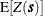is constant and spatial increments define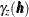—the SRF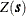is characterized as intrinsically stationary (Cressie 1993, p. 40).

The expected valueis the first statistical moment of the SRF. The second statistical moment of the SRFis the covariance function between two points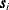and
in, and it is defined as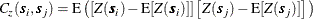When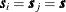, the covariance expression provides the variance at.

The assumption of a constant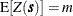means that the expected value is invariant with respect to translations of the spatial location. The covariance is considered invariant to such translations when it depends only on the distance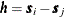between any two pointsand. If both of these conditions are true, then the preceding expression becomes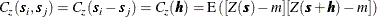When bothand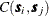are invariant to spatial translations, the SRFis characterized as second-order stationary (Cressie 1993, p. 53).

In a second-order stationary SRF the quantity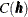is the same for any two points that are separated by distance. Based on the preceding formula, for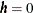you can see that the variance is constant throughout a second-order stationary SRF. Hence, second-order stationarity is a stricter condition than intrinsic stationarity.

Under the assumption of second-order stationarity, the semivariance definition at the beginning of this section leads to the conclusion that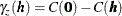which relates the theoretical semivariance and covariance. Keep in mind that the empirical estimates of these quantities are not related in exactly the same way, as indicated in Schabenberger and Gotway (2005, section 4.2.1).